# Basic Data Structures

## Collections

• Like all containers, collections can grow, shrink, or change over time.

• Collections support basic operations such as: insert elements to a collection, remove elements from a collection, or search whether an element belongs to a collection.

# Add an element pointed to by x.
insert(C, x)
# Given a pointer x to an element in a collection C, remove x from C.
remove(C, x)
# Given a collection C and a key k, return a pointer x to an element in C.
# Output: x.key = k or NIL
search(C, k)

## Arrays

An array is a container that can hold a fixed number of items that should be of the same type.

arr = [1, 2, 3, 4, 5, 6, 7, 8, 9, 10]
• Each array item is called an element.

• The location of each element, in an array, can be identified using numerical indices.

• A basic property of an array is its size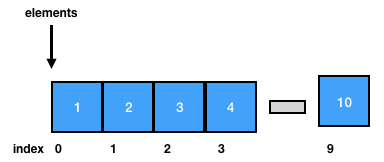## Array insert

def insert(arr, item, k):
if k >= len(arr):
raise Exception
arr[k] = item

return arr
> insert([1,2,3], 4, 1)
[1, 4, 3]

Warning: Arrays in Python are not arrays, but lists internally. This makes the implementation of several array operations look weird.

def append(arr, item):
# Fishy, just for demo purposes
arr = arr + [None]
arr[len(arr) - 1] = item

return arr

## Array remove

def remove(arr, item_index):
if item_index >= len(arr):
raise Exception

return arr[0:item_index] + arr[item_index + 1:len(arr)]

Remove an item by index splits the array in 2, 1 part from the beggining of the array till the removal point, second part from the removal point till the end of the array.

> remove([1,2,3,4,5], 5)
[1, 2, 4, 5, 10]

> remove([1,2,3,4,5,10], 0)
[2, 3, 4, 5, 10]

## Array search and contains

search returns the first position of item in arr

def search(arr, item):
for i in range(len(arr)):
if arr[i] == item:
return i
return -1
> search([1,2,3,4,5], 3)
2

contains returns a Boolean if the provided item exists in an array

def contains(arr, item):
for i in arr:
if i == item:
return True
return False

## Stacks

• Stacks and queues are abstract data types that represent dynamic collections, where the way an element can be removed from a set is prespecified

• LIFO: Last-in, First-out (stacks)
• FIFO: First-in, First-out (queues)
• If a stack consists of elements S[1..S.top], S is the element at the bottom of the stack and S[S.top] is the element at the top of the stack. If S.top = 0, the stack is empty.

## Stack Operations

• The insert operation on a stack is called as push. The remove operation is called as pop.

• The top operation on a stack returns that most recently added element.

• The empty operation checks whether the stack is empty.

## Representation of Stacks using arrays

Stacks are usually implemented using a backing array and a pointer that points to the top of the stack:

class Stack():
def __init__(self, size = -1):
# Stack size, configurable
if size > 0:
self.size = size
else:
self.size = 10
# Backing array
self.arr = map(lambda x: None, range(self.size))
# Top pointer
self.top = 0

We initialize the Stack calling the constructor

> s = Stack(10)
> s.arr
[None, None, None, None, None, None, None, None, None, None]
> s.top
0

## push and pop

push adds an element to a stack.

def push(s, element):
if s.top >= len(s.arr):
raise Exception # StackOveflow

s.arr[s.top] = element
s.top = s.top + 1
return s

> s = push(s, 10)
> s.arr
[10, None, None, None, None, None, None, None, None, None]
> s.top
1

pop removes and returns the top element from the stack

def pop(s):
if s.top == 0:
raise Exception # No element in stack

result = s.arr[s.top - 1]
s.top = s.top - 1
return result

> pop(s)
10
> s.arr
[10, None, None, None, None, None, None, None, None, None]
> s.top
0

## Fun with stacks

One common problem when writing code in languages like Lisp is that we have too many parentheses to check. To solved this problem, we need to implement a “balanced parenthesis checker (bpc)”.

Some Lisp code:

(setf (fdefinition 'f) #'(lambda (a) (block f b...)))

Some example runs:

• bpc("()") -> True
• bpc("(())((())") -> False
def bpc(inp):
pass

## Queues

• In a queue, the element to be removed is the oldest one

• If a queue consists of elements Q[1..n], Q.head points to its head and Q.tail points the next position where a new element can be added to the queue.

When Q.head = Q.tail, it means that the queue is empty.

• The insert operation on a queue is called as enqueue. The remove operation is called as dequeue.

## Representation of Queues Using Arrays

A queue with a backing array is the simplest queue. The main dissadvantage is that it cannot grow dynamically.

class Queue():
def __init__(self, size = -1):
if size > 0:
self.size = size
else:
self.size = 10
# Backing array initialization
self.arr = map(lambda x: None, range(self.size))
# Queue pointers
self.tail = 0

## Adding items with enqueue

def enqueue(self, item):
if self.tail >= len(self.arr):
raise Exception ## Queue full

self.arr[self.tail] = item
self.tail = self.tail + 1
#print "enqueue: [%s] %d" % (', '.join(map(str, self.arr)), self.tail)

The enqueue operation adds the item to the tail position and advances the tail pointer.

> q = Queue(10)
> q.enqueue(4)
> q.enqueue(6)

enqueue: [4, None, None, None, None, None, None, None, None, None] 1
enqueue: [4, 6, None, None, None, None, None, None, None, None] 2

## Removing items with dequeue

def dequeue(self):
if self.tail == 0:
raise Exception ## Queue empty

result = self.arr[self.tail]
self.arr = self.arr[1:len(self.arr)] + [None]
self.tail = self.tail - 1
#print "dequeue: [%s] %d" % (', '.join(map(str, self.arr)), self.tail)
return result

The dequeue operation returns the item at the head position.

> q = Queue(10)
> q.enqueue(4)
> q.enqueue(6)
> q.enqueue(8)
> print q.dequeue()
> print q.dequeue()

enqueue: [4, None, None, None, None, None, None, None, None, None] 1
enqueue: [4, 6, None, None, None, None, None, None, None, None] 2
enqueue: [4, 6, 8, None, None, None, None, None, None, None] 3
dequeue: [6, 8, None, None, None, None, None, None, None, None] 2
4
dequeue: [8, None, None, None, None, None, None, None, None, None] 1
6

## Extra queue operations

How many empty spots are left in the queue?

def capacity(self):
return len(self.arr) - self.tail

Is the queue empty?

  def isempty():
return self.tail == 0

Is the queue full?

  def isfull():
return self.tail == len(self.arr) - 1

## Uses of queues

• To model producer / consumer problems
• In multithreading environments we use blocking and sychronized queues
• To model graph and tree traversals

## Lists

A list is a data structure that can store items (usually) of the same type and in a specific order.

Typically, we can create a list by writing the values (items) we want to store in a comma-separated list.

In Python, a list is an array.

$$1, 2, 3, 4, 5$$

• A linked list is a data structure where objects are arranged in a linear order and this order is determined by poiters attached to each element of the list.

• There are two types of linked lists: doubled and singly.

• Linked lists support all the operations of dynamic collections.

• A doubly linked list L represents a dynamic collection {1, 4, 9, 16}, where the attribute L.heap points to the head of the list and L.tail points the last element of the list (where after this there is no next element). If L.heap = NIL, it means that the list is empty.

• Given that x is an element of a list L, x.next points to the successor element of x and x.prev points to the predecessor element of x.

## More Forms of Linked Lists

• If a list is singly linked, the prev pointer is omitted.

• If a list is ordered, the elements of the list are arranged in linear order. Then, the minimum element is the head of the list, whereas the maxinum element is the tail of the list.

• If a list is cincular, the prev pointer of the head of the list points to its tail, and the next pointer of the tail of the list points to its head.

## Representation of Data Structures in Computer Memory

An array is allocated as one block of memory. Each array element has its own space in the array and it can be directly accessed using the [] syntax. The following figure illustrates how an array might look like in memory.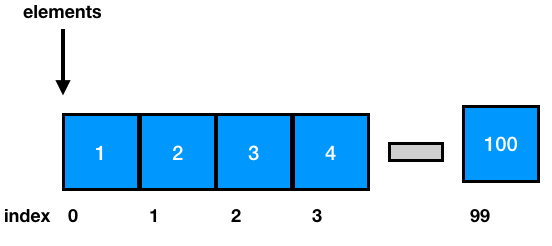The size of the arrays is fixed. This means that this size is known at compile time.

To avoid the above case, we can declare arrays with more capasity, in case we need this in the future.

Even though this strategy can be convenient, we maybe have wasted space in the array. Also, if we finally need to process much more elements than the capacity of the array, the code will break.

Inserting new elements at the front of the array is expensive, because the already stored elements need to be shifted over to make room to the new added elements.

To overcome these problems, we can use linked lists that separately allocate memory for each element, when it is actually necessary.

Contrary to arrays that allocate memory for all its elements together in block of memory, linked lists separately allocate space for each element in its own block of memory called as “linked list element” or “node”.

The list uses pointers to connect all its nodes together (i.e. such as the links in a chain).

Each node has two fields. A “data” field that stores the element and a “next” field, which is the pointer to the next node. Each node is allocated in the heap.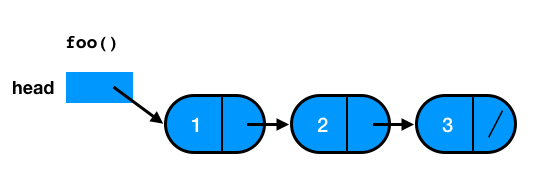## Implementation of a Linked List in Python

In order to implement a linked list in Python, we need to create a Node that will store data and a reference to the next node.

## The Class Node

class Node:
def __init__(self,initdata):
self.data = initdata
self.next = None

def getData(self):
return self.data

def getNext(self):
return self.next

def setData(self,newdata):
self.data = newdata

def setNext(self,newnext):
self.next = newnext

## The New Node

>>> temp = Node(93)
>>> temp.getData()
93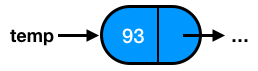## An Unordered List Class

class UnorderedList:

def __init__(self):

def isEmpty(self):

temp = Node(item)
self.head = temp

## The New List

>>> mylist.add(31)
>>> mylist.add(54)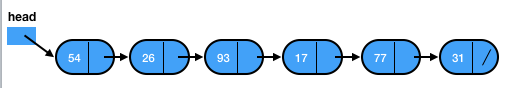• Methods that implement operations, such as size, search, and remove, are based on a technique that is called as linked list traversal.

• Traversal refers to the process of systematically visiting each node of the list. To achieve this, we use an external reference that starts from the first node of the list, and we continue the passage.

• In particular, we visit one node, and then we move the reference to the next node by “traversing” the next reference.

## List Size

• To implement the size method, we need to traverse the linked list, counting the nodes of the list.

• In the beginning, the counter (current) is set to 0, because there is not currely any node.

• We continue checking for new nodes until the current reference reaches the end of the list (None).

def size(self):
count = 0
while current != None:
count = count + 1
current = current.getNext()

return count

## Searching in a List

To implement the search method, we develop the following strategy. We visit each node of the list and we check whether this node has stored the data that we are looking for. We conduct this process until we find the appropriate node.

def search(self,item):
found = False
if current.getData() == item:
found = True
else:
current = current.getNext()

return found

## Search for an Element

>>> mylist.search(17)
True

Here, the traversal needs to move up to the node that contains the element 17.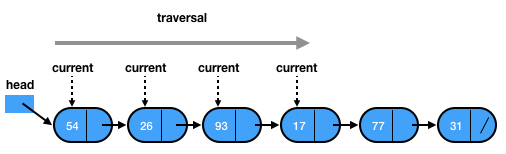## Remove an Element

To implement the remove method, we need to perform two passes.

• First, we traverse the list until we find the element to be removed. This step is similar with the traversal we conduct for the search method.

• Second, we update the nodes that contain the references to the elements. If we remove the node, how can we update the links to the next nodes?

## The previous Reference

Since a linked list does not permit backwards traversal, we use two external references.

The first reference is the current one that represents the current location of the traverse, and the second reference is the previous one that represents a node behind the current.

## The remove Method

def remove(self,item):
previous = None
found = False
if current.getData() == item:
found = True
else:
previous = current
current = current.getNext()

if previous == None:
else:
previous.setNext(current.getNext())

## Sets

In mathematics, a set is a collection of distinct elemets.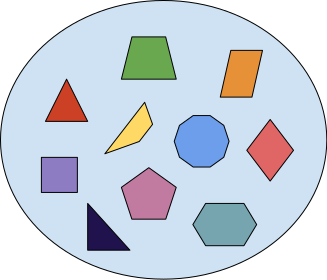## Implement a Set from a List

class Set(object):

def __init__(self, iterable):
data = list(iterable)
data.sort()
result = data[:1]
for elem in data[1:]:
if elem == result[-1]:
continue
# add as it is previously defined in UnorderedList
self.data = result

## Add an Element to a Set

def add(self, elem):
if elem not in self:
# see the insert method presented in the arrays
insert(self.data, elem)

## Remove an Element from a Set

def remove(self, elem):
data = self.data
# return the index of the item; see arrays' search method
i = search(self, elem)
if i<len(data) and data[i] == elem:
del data[i]

## Maps

A map is an abstract data structure that stores key-value pairs and there cannot be duplicate keys.

Such a data structure is also called as: associative array, symbol table, or dictionary.

Maps are usuful in order to:

• store objects to specific keys, and

• lookup of the values associated with a particular key.

## An Example of a Map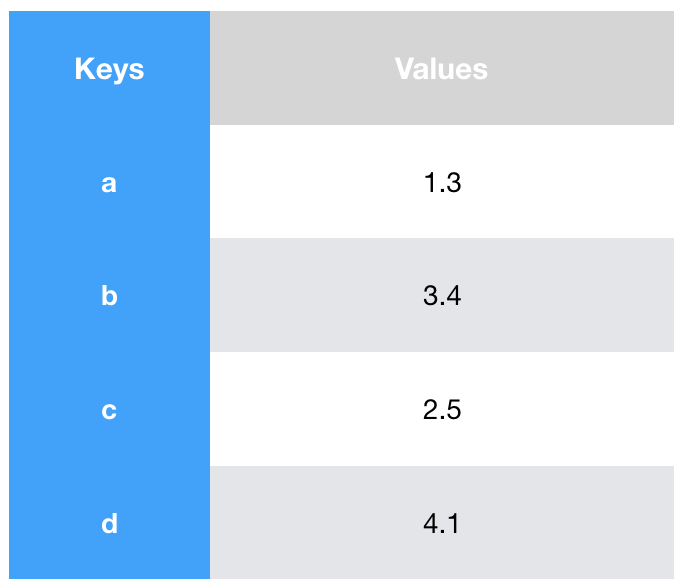## Operations of the Map Data Type

• The method put adds a new key-value pair to a map. If the key is already in the map, it replaces the old value for that key.

• The method get returns the value of that key, or None if there is no value.

• The method delete removes a key-value pair from a map.

## Implementation of a Map

• Arrays

• Binary search trees

• Hash tables

## A Linked List Implementation of a Map

We can store key-value pairs in a linked list as it is illustrated in the following figure.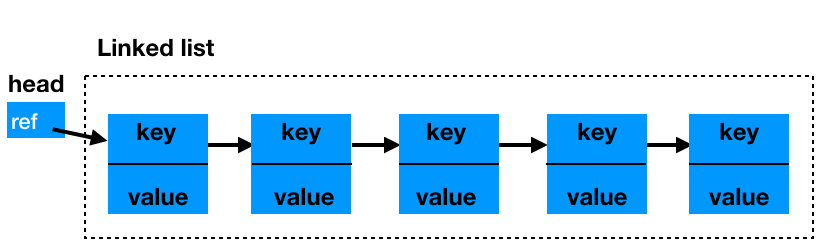## Basic Operations

• get(k): It iterates the list until it finds the item with key k.

• put(k, v): Given Node x = get(k), if x exists, it replaces the value of x with v. Otherwise, it creates a new node(k,v) and adds this at the front of the list.

• remove(k): Given Node x = get(k), if x does not exist, it returns null. Otherwise, it removes node x from the list.

• Complexity: $$O(n)$$ on a map with $$n$$ elements.

## Implementing Maps Using Hashing

• Can we do better in complexity terms?

• Yes, by building a type of a data structure that can search in $$O(1)$$ time. This can be achieved with a concept called as hashing.

## Hash Tables

• A hash table is a collection of elements that are stored in such a way that it is easy for one to find them later.

• The positions of a hash table start from $$0$$ and at each position, it can store an item.

• The mapping between an element and a slot in the collection, where an element belongs to is called hash function. However, we are going to talk in another lecture about it.

## Initialization of a Hash Table

• We are initializing a hash table by adding the special Python None value to the slots of the table.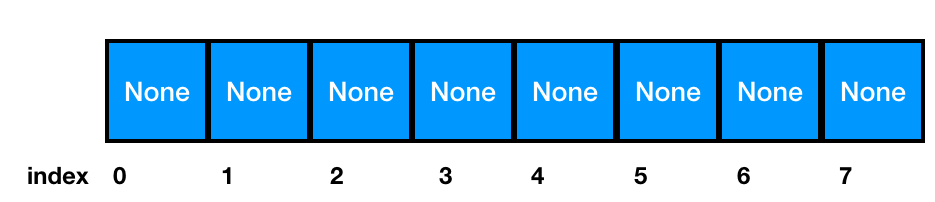## How does it work?

• Assume that we have the set $$S= {54,26,93,17,77,31}$$ and we want to add the items into a hash table of size $$11$$.

• A simple hash function takes an item and divides it by the table size.

• The remainder is the hash value, which will indicate the positions where the items will be inserted in the hash table.

## Implementation of Map in Python

class HashTable:
def __init__(self):
self.size = 11
self.slots = [None] * self.size
self.data = [None] * self.size

## Put Values

def put(self,key,data):
hashvalue = self.hashfunction(key,len(self.slots))

if self.slots[hashvalue] == None:
self.slots[hashvalue] = key
self.data[hashvalue] = data
else:
if self.slots[hashvalue] == key:
self.data[hashvalue] = data  #replace
else:
nextslot = self.rehash(hashvalue,len(self.slots))
while self.slots[nextslot] != None and \
self.slots[nextslot] != key:
nextslot = self.rehash(nextslot,len(self.slots))

if self.slots[nextslot] == None:
self.slots[nextslot]=key
self.data[nextslot]=data
else:
self.data[nextslot] = data #replace

def hashfunction(self,key,size):
return key%size

def rehash(self,oldhash,size):
return (oldhash+1)%size

## Get Values

def get(self,key):
startslot = self.hashfunction(key,len(self.slots))

data = None
stop = False
found = False
position = startslot
while self.slots[position] != None and  \
if self.slots[position] == key:
found = True
data = self.data[position]
else:
position=self.rehash(position,len(self.slots))
if position == startslot:
stop = True
return data

def __getitem__(self,key):
return self.get(key)

def __setitem__(self,key,data):
self.put(key,data)